# pLARmEB: integration of least angle regression with empirical Bayes for multilocus genome-wide association studies

## Abstract

Multilocus genome-wide association studies (GWAS) have become the state-of-the-art procedure to identify quantitative trait nucleotides (QTNs) associated with complex traits. However, implementation of multilocus model in GWAS is still difficult. In this study, we integrated least angle regression with empirical Bayes to perform multilocus GWAS under polygenic background control. We used an algorithm of model transformation that whitened the covariance matrix of the polygenic matrix K and environmental noise. Markers on one chromosome were included simultaneously in a multilocus model and least angle regression was used to select the most potentially associated single-nucleotide polymorphisms (SNPs), whereas the markers on the other chromosomes were used to calculate kinship matrix as polygenic background control. The selected SNPs in multilocus model were further detected for their association with the trait by empirical Bayes and likelihood ratio test. We herein refer to this method as the pLARmEB (polygenic-background-control-based least angle regression plus empirical Bayes). Results from simulation studies showed that pLARmEB was more powerful in QTN detection and more accurate in QTN effect estimation, had less false positive rate and required less computing time than Bayesian hierarchical generalized linear model, efficient mixed model association (EMMA) and least angle regression plus empirical Bayes. pLARmEB, multilocus random-SNP-effect mixed linear model and fast multilocus random-SNP-effect EMMA methods had almost equal power of QTN detection in simulation experiments. However, only pLARmEB identified 48 previously reported genes for 7 flowering time-related traits in Arabidopsis thaliana.

## Introduction

Most complex traits in human, plant and animal genetics are quantitative traits and these traits are controlled by multiple quantitative trait loci (QTLs). The identification of these loci is usually performed by QTL mapping or genome-wide association study (GWAS). A large number of single-nucleotide polymorphisms (SNPs) can be easily obtained for the genotypes by the rapid development of sequencing and genotyping technologies. If all the SNPs are included in a genetic model, the number of SNPs will be much larger than the sample size. The commonly used methods are infeasible for such an oversaturated model.

Many approaches have been proposed to estimate the parameters in the oversaturated model and these approaches include ridge regression (Hoerl and Kennard, 1970), stochastic search variable selection (George and McCulloch, 1993; Yi et al., 2003), Bayesian shrinkage estimation (Meuwissen et al., 2001; Wang et al., 2005), penalized maximum likelihood (Zhang and Xu, 2005; Hoggart et al., 2008; Zhang et al., 2012), empirical Bayes (Xu, 2010) and Bayesian-LASSO (Bayesian-least absolute shrinkage and selection operator; Park and Casella, 2008; Yi and Xu, 2008). However, these methods are mainly proposed for linkage analysis in biparental segregation populations, rather than for GWAS in natural population.

GWAS has been used to dissect the genetic foundation of quantitative traits (Zhang et al., 2005, 2010; Yu et al., 2006; Kang et al., 2008; Zhou and Stephens, 2012; Wang et al., 2016). The widely used approach, such as efficient mixed model association (EMMA; Kang et al., 2008; Zhou and Stephens, 2012), was proposed for single-marker analysis under the population structure and polygenic background controls. However, this method has relatively low power in detecting small-effect QTLs. To overcome these problems, therefore, multilocus model methods have been suggested (Fridley et al., 2010; Lü et al., 2011), for example, a Bayesian-inspired penalized maximum likelihood approach (Zhang and Xu, 2005; Hoggart et al., 2008) and PUMA (Penalized Unified Multiple-locus Association; Hoffman et al., 2013). These methods can be used if the number of variables in the multilocus model is not too large. Recent strategies for high-dimensional modeling have focused on reducing the dimension of a large matrix and then selecting the most potentially associated SNPs by using shrinkage methods such as the LASSO and SCAD (smoothly clipped absolute deviation) penalty (Fan and Lv, 2008; Wu et al., 2009). Although other multilocus approaches have also been proposed by Segura et al. (2012), Moser et al. (2015), Liu et al. (2016), Wang et al. (2016) and Wen et al. (2017), now further refinement and studies are still needed.

In this study, we integrated least angle regression (LARS) algorithm with empirical Bayes to perform multilocus GWAS for quantitative traits, as the LARS algorithm makes LASSO (Tibshirani, 1996) efficient and acceptable (Efron et al., 2004). To control polygenic background, we adopted the model transformation of Wen et al. (2017) that whitens the covariance matrix of the polygenic matrix K and residual noise. The LARS algorithm was implemented on the transformed model to select SNPs that are most potentially associated with the trait, empirical Bayes was used to estimate the effects of all the selected SNPs and all the nonzero effects were further examined by likelihood ratio test so as to confirm true quantitative trait nucleotides (QTNs). We refer to this method as the pLARmEB (polygene-background-control-based least angle regression plus empirical Bayes). pLARmEB was validated by analysis of the data sets from a series of Monte Carlo simulation experiments and seven Arabidopsis flowering time traits. We also discussed the possibility of applying pLARmEB for linkage analysis.

## Materials and methods

### Genetic model

Let yi (i=1,,n) be the phenotypic value of the ith individual in a sample of size n from a natural population. The genetic model is expressed by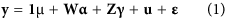where y=(y1, ,yn)T; 1 is a n × 1 vector of 1 and μ is total average; α is population structure effect as fixed; γ~MVNm(0γ) are QTN effects as random,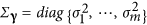and m is the number of putative QTNs; W and Z are the corresponding designed matrices for α and γ; polygenic effects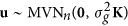is a n × 1 random vector and K is a known n × n relatedness matrix; and ɛ is residual error with an assumed MVNn(0,σ2In) distribution, σ2 is residual error variance and In is an n × n identity matrix.

As γ is treated as being random, the variance of y in the model (1) is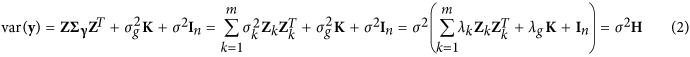where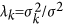(k=1,,m),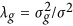and H=Z diag{λ1,,λm}ZT+λgK+In

Using EMMA, we can obtain the estimate of λg, denoted by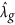. Let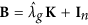, an eigen (or spectral) decomposition of the positive semidefinite matrix B was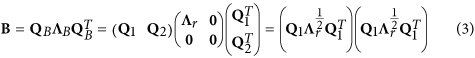where QB is orthogonal, Λr is a diagonal matrix with positive eigenvalues, r=Rank(B), Q1 and Q2 are the n × r and n × (nr) block matrices of QB, respectively, and 0 is the corresponding block zero matrix (Wen et al., 2017).

Let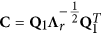, the model (1) is changed to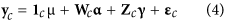where yc=Cy, 1c=C1, Wc=CW, Zc=CZ and ɛc=Cu+~MVNn(0,σ2In) (Wen et al., 2017).

In the above model (4), let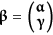, Y=yc1c μ with a zero mean, and standardizing each column in matrix (Wc Zc) produces a new matrix X with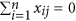and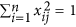(j=1,,m). Therefore, the model (4) can be rewritten as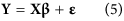### Parameter estimation

#### LARS for the full model

LARS is a flexible method for variable selection that has been described previously (Efron et al., 2004). We used the LARS algorithm to select the n−1 variables that are most likely associated with quantitative trait of interest.

First, let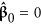, so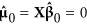Then, suppose that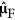is the current LARS estimate and that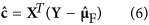is the vector of current correlations. The active set is the set of indices corresponding to covariates with the greatest absolute current correlations,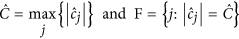Let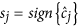for j F. We can calculate XF=(···sjxj···)j F, uF=XFωF,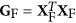,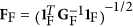, where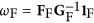, and 1F being a vector of 1 with the length of equaling |F|.

Third, updatein the LARS algorithm: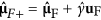where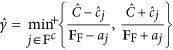, min+ indicates that the minimum is taken over only positive components within each choice of j in the formula of, and aXTuF.

Repeat step 2 to step 3 until a criterion of convergence is satisfied. The above algorithm was conducted by lars package (http://cran.r-project.org/web/packages/lars/) in R language.

Usually, if all the marker effects are included in one genetic model, the parameters cannot be estimated under the situation of mn, where n is sample size and m is the number of variables. As most markers are not likely associated with the trait of interest, once the markers with zero effects are deleted from the full model, marker effects of the reduced model is estimable. In each LARS variable selection, the n−1 SNPs that are most potentially associated with the trait are selected to construct the reduced model.

#### Empirical Bayes estimation in the reduced model

In the reduced model,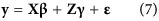where y is the same as that in the model (1); β is a vector of fixed effect, γ is a vector of random effect of the selected markers and X and Z are the design matrices for β and γ, respectively. All the parameters in the model (7) were estimated by empirical Bayes proposed by Xu (2010).

The fixed effect β and residual variance σ2 were estimated by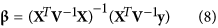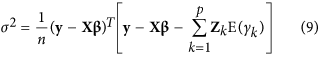where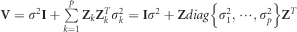. The random effect γk of each marker and its prediction error var(γk) were predicted by best linear unbiased prediction: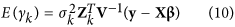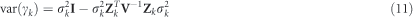where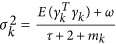, ω=τ=0, and mk is the number of genotypes at locus k. The method requires inverse of matrix V. If the sample size is large, that is, n>p, binomial inverse theorem (Henderson and Searle, 1980) can be used: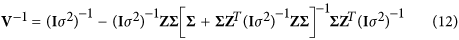where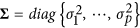Based on our experiences, empirical Bayes is feasible when the number of variables is less than 40 times of the sample size. However, this condition is not frequently met in GWAS. If the LARS algorithm is used to select the variables that are most potentially associated with the trait under polygenic background control, the effects of the selected markers can be estimated by empirical Bayes.

### Likelihood ratio (LR) test

Based on the estimate of marker effect γk in the reduced model, markers with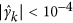are considered not to be associated with the trait; however, the association of the chosen markers with the trait and the effects θ={γ(1),,γ(q)} needs to be tested, where q is the number of SNPs in the reduced model. To test the null hypothesis H0:γ(i)=0, that is, no QTL linked to the marker, we conducted an LR test by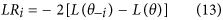where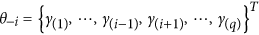,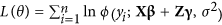is a log-likelihood function, φ(yi;+,σ2) is a normal density function with mean + and variance σ2 and LOD=LR/4.605. The critical value for significance was set at LOD=2.0 (Bu et al., 2015).

### AIC and BIC for testing goodness of fit of models

The goodness of fit for a statistical model can be measured by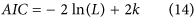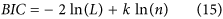where L is the likelihood function value and k is the number of independent variables, and n is sample size. Smaller Akaike information criterion (AIC) or Bayesian information criterion (BIC) value indicates a good fit.

pLARmEB has been implemented in R and its software can be downloaded from https://cran.r-project.org/web/packages/mrMLM/index.html.

### Data sets for analyses

One Arabidopsis data set and four Monte Carlo simulated data sets were used to validate pLARmEB. Each data set contained phenotypic observations for quantitative traits and genotypic values for molecular markers.

#### The Arabidopsis data set

The data set downloaded from http://www.arabidopsis.org/ includes 199 diverse inbred lines each with 216 130 SNPs and 107 traits (Atwell et al., 2010). Among these traits, seven are related to flowering time, including days to flowering under long days, days to flowering under long days with vernalization, days to flowering under short days, days to flowering under short days with vernalization, days to flowering at 10 °C, days to flowering at 16 °C and days to flowering at 22 °C. We analyzed these traits using pLARmEB, EMMA, multilocus random-SNP-effect mixed linear model (mrMLM) and fast multilocus random-SNP-effect EMMA (FASTmrEMMA) methods. The population structure Q matrix and kinship coefficient matrix K between all the pairs of lines were used to control population structure and polygenic background. We also deleted the SNPs with minor allele frequency <10%. When all the markers on one chromosome were in one genetic model, the markers on other chromosomes were used to calculate K matrix as polygenic background control (Rincent et al., 2014; Yang et al., 2014; Wei and Xu, 2016). Here 50 SNPs most potentially associated with the trait are selected to construct the reduced model. This number may vary across different data sets.

#### Data sets from Monte Carlo simulation in natural population

Three Monte Carlo simulation experiments were conducted to validate pLARmEB. The three data sets are the same as those in Wang et al. (2016). In the first experiment, all the SNP genotypes were derived from 216 130 SNPs reported by Atwell et al. (2010) and 2000 SNPs were randomly sampled from each chromosome (Chr.). The positions of these SNPs in the genome were between 11 226 256 and 12 038 776 bp on Chr. 1, between 5 045 828 and 6 412 875 bp on Chr. 2, between 1 916 588 and 3 196 442 bp on Chr. 3, between 2 232 796 and 3 143 893 bp on Chr. 4 and between 19 999 868 and 21 039 406 bp on Chr. 5 (Wang et al., 2016). The sample size was 199, and this was the number of lines in Atwell et al. (2010). Six QTNs were simulated and placed on the SNPs with rare allelic frequency of 0.30. The heritabilities of the QTNs were set as 0.10, 0.05, 0.05, 0.15, 0.05 and 0.05, respectively; their positions and effects are listed in Supplementary Table S1. The total average was set at 10.0 and residual variance was set at 10.0. For each simulated QTN, we counted the number of samples in which the LOD (logarithm (base 10) of odds) exceeded 2.0 (Bu et al., 2015). A detected QTN within 2 kb of the simulated QTN was considered a true QTN. The ratio of the number of such samples to the total number of replicates (1000) represented the empirical power of this QTN. False positive rate (FPR) was calculated as the ratio of the number of false positive effects to the total number of zero effects considered in the full model. To measure the bias of gene effect estimate, mean squared error (MSE)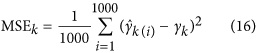was calculated, where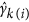is the estimate of effect γk in the ith sample.

We investigated the effect of polygenic background on pLARmEB in the second experiment by adding polygenic effects from a multivariate normal distribution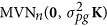, where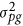is polygenic variance and K is a pairwise kinship coefficient matrix among individuals. Here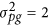, so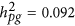. The QTN size (h2), total average, residual variance and other parameter values were the same as those in the first experiment, and all the parameters are listed in Supplementary Table S2.

In the third experiment, we investigated the effect of epistatic background on pLARmEB. Three epistatic QTNs were added. The related parameters for the simulated three epistatic QTNs have been described in Wang et al. (2016). The QTN sizes (h2), total average, residual variance and other parameter values were also the same as those in the first experiment (Supplementary Table S3).

#### Monte Carlo simulation experiments in backcross

To test whether pLARmEB can be used in biparental population, we conducted another simulation experiment. In this experiment, 200 individuals each with 10 001 evenly spaced markers on the entire genome of 100 000 cM length were simulated in backcross population. Eight main-effect QTLs were simulated and placed at marker positions. The sizes and locations of these QTLs are listed in Supplementary Table S4. The population mean (b0) and residual error variance (σ2) were set at 10 and 10, respectively. The number of replicates was set at 200.

## Results

### Monte Carlo simulation studies

#### Statistical power for QTN detection

To validate pLARmEB, three simulation experiments were conducted. In the first experiment, each simulated sample was analyzed by pLARmEB, least angle regression plus empirical Bayes (LARmEB), EMMA, FASTmrEMMA, mrMLM and Bayesian hierarchical generalized linear model (BhGLM). Among the 1000 samples, the first 100 were further analyzed using the BhGLM method. As shown in Supplementary Table S1 and Figure 1a, the average power for the above 6 methods was 77.1, 68.9, 46.0, 70.7, 68.6 and 54.5%, respectively. The method in which polygenic background was controlled had the highest average power among the six methods (Figure 1a). To further confirm the effectiveness of pLARmEB, polygenic effect simulated from multivariate normal distribution (r2=9.2%) was added to each phenotype in the second experiment and three epistatic QTNs (r2=15%) were added in the third simulation experiment. The average powers based on pLARmEB, LARmEB, EMMA, FASTmrEMMA, mrMLM and BhGLM were 78.3, 69.6, 42.5, 75.0, 67.6 and 60.7%, respectively, in the second experiment (Supplementary Table S2); and 74.4, 57.5, 39.1, 59.2, 58.9 and 56.3%, respectively, in the third experiment (Supplementary Table S3). The highest average power was observed when pLARmEB included polygenic background control.

#### Accuracies of estimated QTN effects

MSE measured accuracies of estimated QTN effects, and low MSE indicates high accuracy for parameter estimation. As shown in Figure 1b and Supplementary Tables S1–S3, the average MSEs based on pLARmEB, LARmEB, EMMA, FASTmrEMMA, mrMLM and BhGLM were 0.0895, 0.1005, 0.5432, 0.2885, 0.0940 and 0.2577, respectively, in the first experiment (Figure 1b and Supplementary Table S1); 0.0917, 0.0997, 0.5680, 0.3227, 0.0852 and 1.3139, respectively, in the second experiment (Supplementary Table S2); and 0.0973, 0.1240, 0.5973, 0.3450, 0.1024 and 0.3934, respectively, in the third experiment (Supplementary Table S3). pLARmEB had the highest accuracy for estimating QTN effect among the six methods.

#### FPR and ROC curve

High FPR is a major concern in GWAS. To overcome this issue, a very high significance level was frequently adopted in genome-wide single marker scan. In our multilocus method, a less stringent significance level (LOD=2.0) was recommended. We wanted to know whether this criterion produces high FPR. All the FPR results in the three simulation experiments are listed in Supplementary Tables S1–S3. Clearly, the FPRs based on pLARmEB, LARmEB, EMMA, FASTmrEMMA, mrMLM and BhGLM were 0.0009, 0.0127, 0.0325, 0.0084, 0.0168 and 0.0115 (%), respectively, in the first experiment (Supplementary Table S1); 0.0025, 0.0010, 0.0166, 0.0081, 0.0210 and 0.0093%, respectively, in the second experiment (Supplementary Table S2); and 0.0089, 0.0031, 0.0253, 0.0148, 0.0265 and 0.0120%, respectively, in the third experiment (Supplementary Table S3). These results indicate that pLARmEB had a low FPR.

To compare various approaches for their efficiencies in the detection of significant QTNs, receiver operating characteristic (ROC) curve was plotted. ROC is a plot of average power against FPR. We calculated the corresponding average powers for the 41 thresholds between 10−6 and 10−2 in the first simulation experiment, and compared the ROC curves among the above 6 methods. Under the 0.01 to 0.001 significant levels, pLARmEB has the highest power to detect QTN among the six methods (Figure 2).

#### Computational efficiency

We scanned and identified SNPs that were associated with the trait on each chromosome using LARS. We then included all the potentially associated SNPs across the genome into one genetic model and estimated their effects by empirical Bayes (Xu, 2010). For the first simulation experiment, the above procedures took 4.20, 6.82, 68.77, 8.32, 13.29 and >100 h (Intel Core i5-4570 CPU 3.20 GHz, Memory 7.88G, Nanjing, China) for pLARmEB, LARmEB, EMMA, FASTmrEMMA, mrMLM and BhGLM, respectively. pLARmEB took the least computing time among the six approaches. A similar trend was found in real data analyses (Supplementary Table S5).

### Analysis of the Arabidopsis data set

To test the performance of pLARmEB, a data set containing 7 Arabidopsis flowering traits along with 216 130 SNPs in Atwell et al. (2010) were reanalyzed by pLARmEB, EMMA, FASTmrEMMA and mrMLM. All the significantly associated SNPs were used to fit the regression for each trait and model fitness was reflected by AIC and BIC values. The AIC values for all the seven traits based on pLARmEB were much lower than those based on EMMA, FASTmrEMMA and mrMLM (Table 1). Hence, FASTmrEMMA and mrMLM were better than EMMA and a similar result was also observed from the BIC values. The finding suggests that pLARmEB is better in model fit than EMMA, FASTmrEMMA and mrMLM.

Within 20 kb of each SNP significantly associated with traits, we mined candidate genes for these traits. Among the genes identified in previous studies, pLARmEB, FASTmrEMMA and mrMLM identified more previously reported genes than EMMA (Supplementary Table S6). For example, pLARmEB, FASTmrEMMA and mrMLM identified more than three genes for long days with vernalization, whereas EMMA detected only one gene (AT5G45890). A similar trend was also observed for other traits (Supplementary Table S6). Among these previously reported genes, 48 were identified only by pLARmEB (Table 2). Interestingly, genes AT2G19690 and AT2G19760 identified by pLARmEB were associated simultaneously with long days with vernalization and short days with vernalization SDV, and three genes (AT2G07020, AT2G07040 and AT2G07050) adjacent to the SNP at 2 910 430 bp of chromosome 2 were found to be associated with short days.

## Discussion

Analysis of one random sample in the first Monte Carlo simulation experiment using LARS, empirical Bayes and pLARmEB showed that LARS identified many QTNs with small effects in addition to all the simulated QTNs, and thus its FPR was high (Figure 3a). The empirical Bayes was also able to identify simulated and small-effect QTNs although FPR was decreased (Figure 3b), and pLARmEB detected almost all the simulated QTNs and the effects of nonsimulated QTNs were almost close to zero (Figure 3c). More importantly, 48 previously reported genes in Arabidopsis were identified only by pLARmEB. Therefore, pLARmEB is a good alternative method for multilocus GWAS.

Although pLARmEB was proposed for GWAS, it is appropriate for mapping populations of backcross, doubled haploid and recombinant inbred lines. To illustrate the effectiveness of pLARmEB, pseudo-markers in every d cM were created genome-wide, and the fourth Monte Carlo simulation experiment with 200 simulated data sets was conducted and analyzed using pLARmEB and empirical Bayes. The higher power for QTL detection and less bias for the QTL-effect estimates were observed from pLARmEB than from empirical Bayes (Supplementary Table S4). pLARmEB is also suitable for a population consisting of chromosome segment substitution lines. However, we can only scan marker positions, because we cannot calculate conditional probabilities of pseudo-marker positions. If the number of genotypes in a mapping population is more than two, for example, AA, Aa and aa in F2, the current method requires some modifications.

Among the previously identified genes in Arabidopsis (Supplementary Table S6), a few were found commonly by several approaches and this is different from linkage analysis. The main reason is that GWAS mapping population has a complicated population structure. Although pLARmEB, FASTmrEMMA, and mrRMLM had similar powers of QTN detection in the simulation experiments, different previously reported genes were detected in real data analysis. For example, 48 previously reported genes were identified only by pLARmEB (Table 2). For this reason, we recommend pLARmEB as an alternative method for GWAS and also recommend the joint implementation of several methods in the GWAS analyses of one trait.

The AIC or BIC values of FASTmrEMMA in Wen et al. (2017) and mrRMLM in Wang et al. (2016) are different from the corresponding values in this study. In this study, we considered population structure in GWAS. With the inclusion of population structure in genetic model, some different SNPs are found to be significantly associated with the trait. The above two differences result in different AIC or BIC values for the same trait in different studies.

Multilocus GWAS has become the state-of-the-art GWAS procedure. Iwata et al. (2007, 2009) developed multilocus Bayesian GWAS approaches for quantitative and ordinal traits, although running time is a major concern. Segura et al. (2012) proposed a multilocus linear mixed model method that is simple, stepwise mixed model regression with forward inclusion and backward elimination. Wang et al. (2016) suggested mrMLM and Wen et al. (2017) proposed FASTmrEMMA. To make assumptions more suitable to a given data set, Zhou et al. (2013) and Moser et al. (2015) proposed a hybrid method of mixed linear model and sparse regression model, named Bayesian sparse linear mixed model. In this study, the integration of LARS with empirical Bayes under polygenic background control provides one simple and efficient way for multilocus GWAS. In Arabidopsis real data analysis, the number of SNPs was >1000 times larger than sample size and we were able to scan each chromosome by LARS and include all the associated SNPs across the genome in the multilocus model and estimate their effects by empirical Bayes, and thus pLARmEB is better than EMMA.

To obtain low FPR in GWAS, a relatively stringent significance criterion is widely adopted, such as Bonferroni correction. Even after using a less stringent significance criterion (such as LOD=2.0), pLARmEB has less FPR and higher power than EMMA. We also conducted GEMMA (Zhou and Stephens, 2012) and its power is same as that of EMMA (results not shown). pLARmEB works better than all the other methods considered.

## References

1. Atwell S, Huang YS, Vilhjálmsson BJ, Willems G, Horton M, Li Y et al. (2010). Genome-wide association study of 107 phenotypes in a common set of Arabidopsis thaliana inbred lines. Nature 465: 627–631.

2. Bu SH, Zhao XW, Yi C, Wen J, Tu JX, Zhang YM . (2015). Interacted QTL mapping in partial NCII design provides evidences for breeding by design. PLoS One 10: e0121034.

3. Efron B, Hastie T, Johnstone I, Tibshirani R . (2004). Least angle regression. Ann Statist 32: 407–451.

4. Fan J, Lv J . (2008). Sure independence screening for ultrahigh dimensional feature space. J R Stat Soc Ser B 70: 849–911.

5. Fridley BL, Serie D, Jenkins G, White K, Bamlet W, Potter JD et al. (2010). Bayesian mixture models for the incorporation of prior knowledge to inform genetic association studies. Genet Epidemiol 34: 418–426.

6. George EI, McCulloch RE . (1993). Variable selection via Gibbs sampling. J Am Stat Assoc 88: 881–889.

7. Henderson HV, Searle SR . (1980) On Deriving the Inverse of a Sum of Matrices. Biometrics Unit, Cornell University: Ithaca, New York. Paper No. BU-647-M in the Biometrics Unit Series.

8. Hoerl AE, Kennard RW . (1970). Ridge regression: biased estimation for non-orthogonal problems. Technometrics 12: 55–67.

9. Hoggart CJ, Whittaker JC, De Iorio M, Balding DJ . (2008). Simultaneous analysis of all SNPs in genome-wide and re-sequencing association studies. PLoS Genet 4: e1000130.

10. Hoffman GE, Logsdon BA, Mezey JG . (2013). PUMA: a unified framework for penalized multiple regression analysis of GWAS data. PLoS Comput Biol 9: e1003101.

11. Iwata H, Uga Y, Yoshioka Y, Ebana K, Hayashi T . (2007). Bayesian association mapping of multiple quantitative trait loci and its application to the analysis of genetic variation among Oryza sativa L. germplasms. Theor Appl Genet 114: 1437–1449.

12. Iwata H, Ebana K, Fukuoka S, Jannink JL, Hayashi T . (2009). Bayesian multilocus association mapping on ordinal and censored traits and its application to the analysis of genetic variation among Oryza sativa L. germplasms. Theor Appl Genet 118: 865–880.

13. Kang HM, Zaitlen NA, Wade CM, Kirby A, Heckerman D, Daly MJ et al. (2008). Efficient control of population structure in model organism association mapping. Genetics 178: 1709–1723.

14. Liu X, Huang M, Fan B, Buckler ES, Zhang Z . (2016). Iterative usage of fixed and random effect models for powerful and efficient genome-wide association studies. PLoS Genet 12: e1005767.

15. Lü HY, Liu XF, Wei SP, Zhang YM . (2011). Epistatic association mapping in homozygous crop cultivars. PLoS One 6: e17773.

16. Meuwissen THE, Hayes BJ, Goddard ME . (2001). Prediction of total genetic value using genome-wide dense marker maps. Genetics 157: 1819–1829.

17. Moser G, Lee SH, Hayes BJ, Goddard ME, Wray NR, Visscher PM . (2015). Simultaneous discovery, estimation and prediction analysis of complex traits using a Bayesian mixture model. PLoS Genet 11: e1004969.

18. Park T, Casella G . (2008). The Bayesian Lasso. J Am Stat Assoc Theor Methods 103: 681–686.

19. Rincent R, Moreau L, Monod H, Kuhn E, Melchinger AE, Malvar RA et al. (2014). Recovering power in association mapping panels with variable levels of linkage disequilibrium. Genetics 197: 375–387.

20. Segura V, Vilhjálmsson BJ, Platt A, Korte A, Seren Ü, Long Q et al. (2012). An efficient multi-locus mixed-model approach for genome-wide association studies in structured populations. Nat Genet 44: 825–830.

21. Tibshirani R . (1996). Regression shrinkage and selection via the Lasso. J R Stat Soc Ser B 58: 267–288.

22. Wang H, Zhang YM, Li X, Masinde GL, Mohan S, Baylink DJ et al. (2005). Bayesian shrinkage estimation of quantitative trait loci parameters. Genetics 170: 465–480.

23. Wang SB, Feng JY, Ren WL, Huang B, Zhou L, Wen YJ et al. (2016). Improving power and accuracy of genome-wide association studies via a multi-locus mixed linear model methodology. Sci Rep 6: 19444.

24. Wei JL, Xu S . (2016). A random-model approach to QTL mapping in multiparent advanced generation intercross (MAGIC) populations. Genetics 202: 471–486.

25. Wen YJ, Zhang H, Ni YN, Huang B, Zhang J, Feng JY et al. (2017). Methodological implementation of mixed linear models in multi-locus genome-wide association studies. Brief Bioinform e-pub ahead of print 1 February 2017 doi:10.1093/bib/bbw145.

26. Wu TT, Chen YT, Sobel E, Lange K . (2009). Genome-wide association analysis by lasso penalized logistic regression. Bioinformatics 25: 714–721.

27. Xu S . (2010). An expectation–maximization algorithm for the Lasso estimation of quantitative trait locus effects. Heredity 105: 483–494.

28. Yang J, Zaitlen NA, Goddard ME, Visscher PM, Price AL . (2014). Advantages and pitfalls in the application of mixed-model association methods. Nat Genet 46: 100–106.

29. Yi N, Xu S . (2008). Bayesian LASSO for quantitative trait loci mapping. Genetics 179: 1045–1055.

30. Yi N, George V, Allison DB . (2003). Stochastic search variable selection for identifying quantitative trait loci. Genetics 164: 1129–1138.

31. Yu J, Pressoir G, Briggs WH, Vroh BiI, Yamasaki M, Doebley JF et al. (2006). A unified mixed-model method for association mapping that accounts for multiple levels of relatedness. Nat Genet 38: 203–208.

32. Zhang J, Yue C, Zhang YM . (2012). Bias correction for estimated QTL effects using the penalized maximum likelihood method. Heredity 108: 396–402.

33. Zhang YM, Mao Y, Xie C, Smith H, Luo L, Xu S . (2005). Mapping quantitative trait loci using naturally occurring genetic variance among commercial inbred lines of maize (Zea mays L.). Genetics 169: 2267–2275.

34. Zhang YM, Xu S . (2005). A penalized maximum likelihood method for estimating epistatic effects of QTL. Heredity 95: 96–104.

35. Zhang Z, Ersoz E, Lai CQ, Todhunter RJ, Tiwari HK, Gore MA et al. (2010). Mixed linear model approach adapted for genome-wide association studies. Nat Genet 42: 355–360.

36. Zhou X, Stephens M . (2012). Genome-wide efficient mixed-model analysis for association studies. Nat Genet 44: 821–824.

37. Zhou X, Carbonetto P, Stephens M . (2013). Polygenic modeling with Bayesian sparse linear mixed models. PLoS Genet 9: e1003264.

## Acknowledgements

This work was supported by the National Natural Science Foundation of China (31301229, 31571268), and Huazhong Agricultural University Scientific and Technological Self-innovation Foundation (Program No. 2014RC020).

## Author information

Authors

### Corresponding author

Correspondence to Y-M Zhang.

## Ethics declarations

### Competing interests

The authors declare no conflict of interest.

Supplementary Information accompanies this paper on Heredity website

## Rights and permissions

Reprints and Permissions

• ### Genome-wide association studies and whole-genome prediction reveal the genetic architecture of KRN in maize

• Yixin An
• , Lin Chen
• , Yong-Xiang Li
• , Chunhui Li
• , Yunsu Shi
• , Dengfeng Zhang
• , Yu Li
•  & Tianyu Wang

BMC Plant Biology (2020)

• ### Decryption of favourable haplotypes and potential candidate genes for five fibre quality properties using a relatively novel genome-wide association study procedure in upland cotton

• Junji Su
• , Caixiang Wang
• , Delong Yang
• , Chunhui Shi
• , Ai Zhang
• , Qi Ma
• , Juanjuan Liu
• , Xianliang Zhang
• , Long Huang
•  & Xiongfeng Ma

Industrial Crops and Products (2020)

• ### Identification of quantitative trait nucleotides and candidate genes for soybean seed weight by multiple models of genome-wide association study

• Benjamin Karikari
• , Zili Wang
• , Yilan Zhou
• , Wenliang Yan
• , Jianying Feng
•  & Tuanjie Zhao

BMC Plant Biology (2020)

• ### Multi-Locus GWAS of Quality Traits in Bread Wheat: Mining More Candidate Genes and Possible Regulatory Network

• Yang Yang
• , Yongmao Chai
• , Xuan Zhang
• , Shan Lu
• , Zhangchen Zhao
• , Di Wei
• , Liang Chen
•  & Yin-Gang Hu

Frontiers in Plant Science (2020)

• ### Identification of QTNs Controlling 100-Seed Weight in Soybean Using Multilocus Genome-Wide Association Studies

• Zhongying Qi
• , Jie Song
• , Kaixin Zhang
• , Shulin Liu
• , Xiaocui Tian
• , Yue Wang
• , Yanlong Fang
• , Xiyu Li
• , Jiajing Wang
• , Chang Yang
• , Sitong Jiang
• , Xu Sun
• , Zhixi Tian
• , Wenxia Li
•  & Hailong Ning

Frontiers in Genetics (2020)

## Search